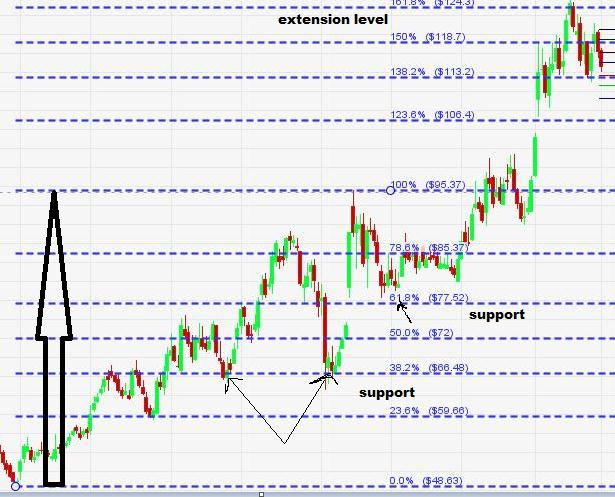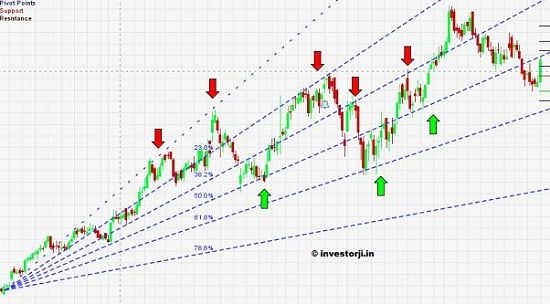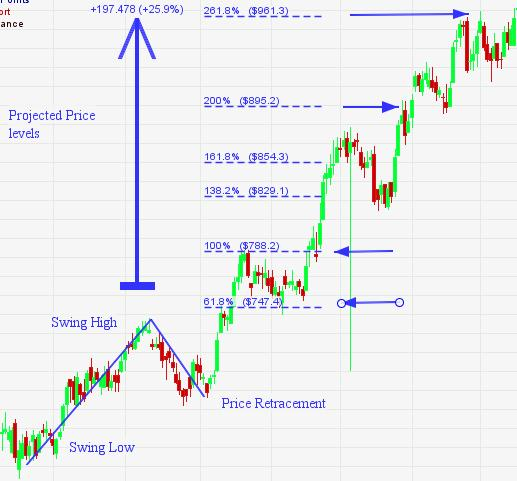View : 285 Click : 0December 3, 2013

How to Use Fibonacci Numbers in Trading & Technical Analysis

December 3, 2013

Fibonacci numbers are widely used in technical analysis. Lots of tools based on Fibonacci numbers are used in the study of charts like Fibonacci retracement, Fibonacci extensions , Fibonacci fans, projections etc.

In this post we will look at what actually are Fibonacci numbers and how they can be used in technical analysis for making trading decisions.

What are Fibonacci numbers ?

Fibonacci series begins with 0 and 1 . The new numbers are obtained by adding the previous number to the current number. so the Fibonacci series can be generated as

0,1,0+1,1+1,2+1,3+2…

= 0, 1, 1, 2, 3, 5, 8, 13 ,21, 34……

Fibonacci Golden Ratios

The above series tends as asymptotically(approaching slower and slower) towards constant ratio.  If we divide each number by its next number we get a constant ratio.

NumberDivided byRatio
8130.615
13210.619
21340.618
34550.618
55890.618
891440.618

If we divide each number by its preceding number we get a fixed ration which approaches 1.618 . For example 13 divide by 8 , 21 divided by 13 , 34 divided by 21 etc. all gives 1.618

NumberDivided byRatio
1381.625
21131.615
34211.619
55341.617
89551.618
144891.618

On dividing every alternate number in forward and backward direction, we also get a fixed ratio.

NumberDivided byRatio
34890.382
892330.382
551440.382
34132.615
89342.618
144552.618

Similarly on dividing the every third number forward and backward we get we get 0.236  and 4.236

These ratios occurs  very frequently in nature because of which they are called as golden ratio. Petals on flowers,  branches on trees, spirals in fruits and vegetables, The eyes, fins and tail of the dolphin follows a Fibonacci number along the body, bones arrangement in  the human body, Even our Human Genome in DNA has a structure which fits into this ratios.  These golden ratio also occurs   frequently in nature. For example wave patterns which emerge from the ocean, growth of a tree or a sunflower, population growth of various species etc.

How Fibonacci numbers are used in Technical analysis

In Technical Analysis, a trend is found to pull back to common Fibonacci ratios, and then continuing back in the usual trend. Several other Fibonacci patterns are used widely in technical analysis and is visible in all the markets. The ratios are used to find the support and resistance levels.  They are also employed in predicting the time frame for the price levels. The support, resistance and target levels can be predicted using Fibonacci ratios in technical analysis.

In technical analysis these ratios are used in percentage terms. The commonly used Fibonacci percentage are 23.6 , 38.2 , 50 , 61.8,  78.6  127.2 , 161.8 and 261.8 % .

Using Fibonacci retracements and extensions

The golden ratio serve as support and resistance level. Buying can be done at Fibonacci support level and selling can be done at a resistance level. Fibonacci retracements and extension levels are calculated using the recent high and low. You can calculate them manually or in a excel sheet. Also every charting software provides Fibonacci tools.

In the below chart of Adani Enterprise you can see how 38.2% and 50% Fibonacci retracement level acting as resistance.In the another chart you can see the Fibonacci support levels. On breaking the Resistance the price moved till 161.8% extension level.Using Fibonacci Fan Lines

Fibonacci fan lines are the lines based on Fibonacci ratios. These fan lines are used to estimate the support and resistance levels or potential reversal zones. The marked arrows in the below chart show the support and resistance levels  obtained through the Fibonacci fan.Using Fibonacci Time Zone

Fibonacci Time zones are vertical lines based on Fibonacci numbers. These lines are used to estimate reversal based on elapsed time. In the below chart you can see the vertical lines showing the reversal timings.Using Fibonacci Projections

Fibonacci projections are also known as Fibonacci extensions. They are used to project the future price levels. To draw Fibonacci projection recent swing low, swing high and price retracement against the swing high are used.Note: One should understand here that it is not necessary that every Fibonacci support or resistance should really work as support or resistance. Trading position should me made only when these Fibonacci levels are confirmed with other technical tool like candlestick reversal pattern , momentum indicators etc.

This site uses Akismet to reduce spam. Learn how your comment data is processed.